# １５　Quantum Mechanics　#universe #mechanics

１５　Quantum Mechanics　#universe #mechanics

１５−１　What is Quantum mechanics？
○Word root：Energy, length, speed, and time, those physical quantities have the smallest unit(quanta) that can not be divided any more.

○About the particles like the size of electron (: 2.8 × 10 ^ -15m), you can see the state (the value of the energy), if you get the wave function by solving the wave equation (Schrodinger equation: partial differential equation 1926) , or if you solve Heisenberg`s equation (the determinant 1925) .
：By differentiating the wave function, we know the values of energy and momentum.
※The wave equation and Heisenberg`s equation is mathematically equivalent.
Since Heisenberg`s equation is more complicated than the wave equation, computation of concrete cases often uses wave equations. On the other hand, when dealing with the general theory of quantum mechanics Heisenberg`s equation is more suitable.

○The Wave equation
• The Wave equation is a Fundamental equation of quantum mechanics. It is contrasted with Newton's equation of motion of classical mechanics.
・We can calculate the state of the matter wave stochastically by the equation.
・The Wave equation cannot be obtained strictly by using classical mechanics or mathematics. It is the equation that successfully describes the physical phenomena.
※Relativity is classical mechanics.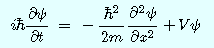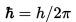： reduced Planck constant
ｔ：time
ｍ： mass of particle
Ψ（Psi）：wave function： Complex quantity, which was introduced to represent the wave
X：position
V： potential energy

○The squared modulus of the wave function is a real number interpreted as the probability density of measuring a particle's being detected at a given place.
・The figure below shows the graph of the probability density and the energy state of matter.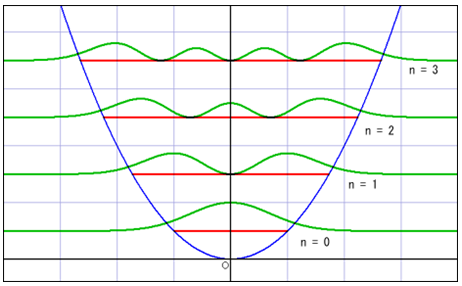※The red line is the energy state(n). The probability density and n is changed at intervals.

☆The universe had particles that have exactly the same properties without limit, at energy following the mathematical rules!

○The universe was born from a quantum fluctuation.

○Wave function is a function of the complex because the wave equation contains complex number. 
・・・The universe is represented by imaginary!

１５−２　Material Wave（de Broglie wave）
○On a submicroscopic level, there is no boundary strict between particles and wave.
→When existence is regarded as a wave motion, in physics, it is called a "place." For example, light is wave motion of an electric field and magnetic field.

※ It is under a very microscopic situation like an electron beam that the character as a wave is actually observed.
※ It is impossible to determine the size of the electron.
※ And the length of orbit around the nucleus is an integer multiple of the wavelength (10 minus 10 square m).
＝Why the electrons are not getting stuck in the nucleus.

○The image of the wave of complex number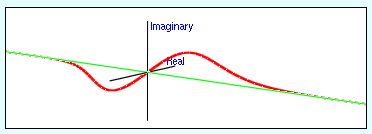※Whole wave represents one of the particles.
※Height and depth of the wave corresponds to the size of the wave function.
：de Broglie wave＝the wave function

○Material Wave：A wave that moves the space represented by the complex number. ：without direction（Scalar wave）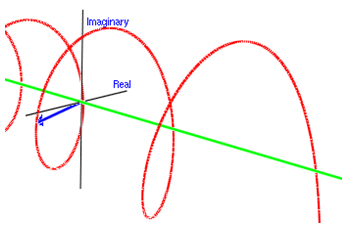○In general, the substance obviously shows the property of waves is the world of elemental particles such as electrons composing atoms, protons and neutrons, the world of less than 10 millionths of a millimeter (10 ^ -10 m).
This is one tenth of a world of nanometer (one millionth of a millimeter (10 ^ - 9 m)) called nanotechnology etc.
And when becoming a world equal to or larger than an atom, the property as a wave hardly appears.

• However, in the world beyond atoms, the nature of waves may appear.
・One example is the superfluid phenomenon of helium at cryogenic temperature. This is called "Bose-Einstein condensation", and the waves of a plurality of atoms overlap each other at cryogenic temperature, thereby exhibiting properties like Bose particles, and the viscosity becomes zero.
※Superfluid refers to a state in which the viscous resistance of a liquid disappears at a constant flow rate or less. There are strange phenomena, such as the liquid climbing the wall by the attraction of the container atom and flowing out from the narrow gap which can not be passed by ordinary liquid.
※Fermi particles (electrons, protons, neutrons) follow Pauli exclusion principle , but Bose particles (photons) do not follow.
・In 1999, the group of Vienna University succeeded to let C60 molecules with 60 carbon atoms gather in the form of soccer balls to draw interference fringes.

１５−３　Applications 
・Description of the behaviors of the subatomic particles
・Computational chemistry
・The presence description of antimatter and negative energy
・Basic theory of semiconductor engineering　→　Electronic devices design
（the laser, the transistor (and thus the microchip), the electron microscope, and magnetic resonance imaging (MRI) , the light emitting diode, USB drives）
・Basic theory of superconductivity and superfluidity
・Quantum cryptography
Quantum computing
・Quantum teleportation
・Parallel universes

○Unsolved problem
・Quantum gravity theory is incomplete.

１５−４　Uncertainty Principle　→　Ｎｏ．１６

【References】
1. 広江克彦”EMANの量子力学” Katsuhiko Hioe” EMAN’s Quantum mechanics”(Japanese)
2.Lecture 3: The Wave Function - MIT OpenCourseWare
3. EMAMの物理学・量子力学調和振動子 EMAN’s Physics ・Quantum mechanics／ harmonic oscillator (Japanese)
4. Quantum mechanics - Wikipedia

【Change log】